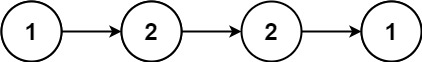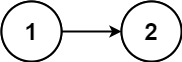# GeetCode Hub

Given the `head` of a singly linked list, return `true` if it is a palindrome.

Example 1:```Input: head = [1,2,2,1]
Output: true
```

Example 2:```Input: head = [1,2]
Output: false
```

Constraints:

• The number of nodes in the list is in the range `[1, 105]`.
• `0 <= Node.val <= 9`

Follow up: Could you do it in `O(n)` time and `O(1)` space?

/** * Definition for singly-linked list. * public class ListNode { * int val; * ListNode next; * ListNode() {} * ListNode(int val) { this.val = val; } * ListNode(int val, ListNode next) { this.val = val; this.next = next; } * } */ class Solution { public boolean isPalindrome(ListNode head) { } }